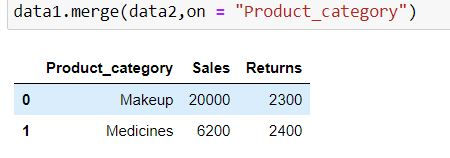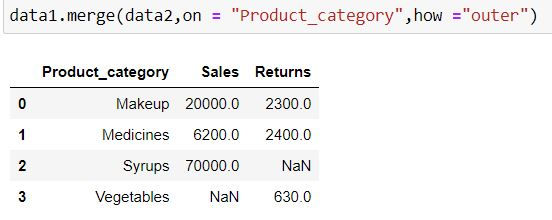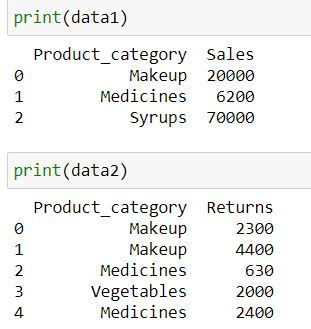top of page
Search
• Ekta Aggarwal

# Merging data in Python

In this article we will learn about the joins available in Python and how to implement them!

There are 5 types of joins available in Python and we shall learn about them in detail.

Special cases like:

Let us firstly import pandas library:

`import pandas as pd`

Basic Syntax:

To join 2 datasets we use .merge( ) function from pandas. The syntax is:

```data1.merge(data2,
on = ["list of common columns to be used for joining "] ,
how = " ",
left_on = ["Column name to be used for joining in left table"] ,
right_on = ["Column name to be used for joining in right table"])```

how : Specifying the type of join : left, right, inner or outer.

Dataset:

Let us consider 2 datasets data1 containing sales and data2 containing returns for various product categories

```data1 = pd.DataFrame({
'Product_category': ['Makeup','Medicines','Syrups'],
'Sales': [20000,6200,70000]})
data2 = pd.DataFrame({
'Product_category': ['Makeup','Vegetables','Medicines'],
"Returns" : [2300,630,2400]})```### Inner Join

Inner Join takes common elements in both the tables and join them. By default pd.merge( ) returns inner join as output.

`data1.merge(data2,on = "Product_category")`

alternatively, you can specify how = "inner"

`data1.merge(data2,on = "Product_category",how ="inner")`Output Explanation: Since both the data have only 2 product categories in common : Makeup and medicines thus we have only 2 rows in our output.

### Left Join

Left join takes all the records from left table (specified before merge keyword) irrespective of availability of record in right table (specified inside parenthesis of merge)

If the record is not available in the right table then missing values get generated for such records.

`data1.merge(data2,on = "Product_category",how ="left")`

Output Explanation: In data1 (left table) we have 3 product categories available thus we have only those 3 categories available in output. Since there is no information about Syrups in data2 (right table) thus we have NaN (missing values) generated for Returns for it.### Right Join

Rihgt join takes all the records from right table (specified inside parenthesis of merge) irrespective of availability of record in left table (specified before merge keyword)

If the record is not available in the left table then missing values get generated for such records.

`data1.merge(data2,on = "Product_category",how ="right")`

Output Explanation: In data2 (right table) we have 3 product categories available thus we have only those 3 categories available in output. Since there is no information about Vegetables in data1 (left table) thus we have NaN (missing values) generated for Sales for it.### Outer Join or Full Join

Outer join or Full join takes all the records from both the tables. Missing values get generated if a record is available in only one table.

`data1.merge(data2,on = "Product_category",how ="outer")`

Output Explanation: In data1 (left table) and data2 (right table) we have 5 unique product categories available thus we have 5 categories available in output.

Since there is no information about Syrups in data2 (right table) thus we have NaN (missing values) generated for Returns for it.

Similarly there is no Sales information available for Vegetables.## Anti - Join

Anti join comprises of those records which are present in one table but not in other.

Let us firstly understand what is indicator =True means in merge( ) function:

`data1.merge(data2, on = "Product_category",how = "outer",indicator=True)`

In _merge column following the meaning of its values:

both: Record is available in both the tables

left_only: Record is available only in left table(data1)

right_only: Record is available only in right table (data2)Let us save this result in a new dataset called merged

`merged = data1.merge(data2, on = "Product_category",how = "outer",indicator=True)`

Anti join 1:

In the following code we are fetching those rows which belong to DATA1 and are not present in DATA2 by filtering for rows where _merge column has values: "left_only"

`merged.loc[merged["_merge"] == "left_only",:]`Output Explanation: In data1 (left table) Syrups is present whose information is absent in data2 (right table) thus we have only that row in the output.

Anti join 2:

In the following code we are fetching those rows which belong to DATA2 and are not present in DATA1 by filtering for rows where _merge column has values: "right_only"

`merged.loc[merged["_merge"] == "right_only",:]`

Output Explanation: In data2(right table) Vegetables is present whose information is absent in data1 (left table) thus we have only that row in the output.### When other columns which are not used for joining have same names.

Let us consider 3 datasets where we have sales for 3 different months:

```Jan_data = pd.DataFrame({
'Product_category': ['Makeup','Medicines','Syrups'],
'Sales': [20000,6200,70000]})

Feb_data = pd.DataFrame({
'Product_category': ['Makeup','Vegetables','Medicines'],
"Sales" : [2300,630,2400]})

Mar_data = pd.DataFrame({
'Product_category': ['Makeup','Vegetables','Books'],
'Sales': [14000,23000,14000]})```

Let us try to join data for Jan and Feb by product category. But wait - In both the datasets we have Sales as another common column. To indicate which column belongs to Jan and which to Feb we can add a suffix to these columns using suffix = option.

First suffix is for the left table, and second for right table.

`Jan_data.merge(Feb_data,on = "Product_category",how ="outer",suffixes=("_Jan","_Feb"))`### Merging more than 2 datasets:

Let us try to merge 3 datasets: Jan, Feb and March data using one single command:

In the following code, Python firstly merges Jan and Feb data, and after that we have specified .merge( ) command by which March data is joined.

```Jan_data.merge(Feb_data,on = "Product_category",how ="outer")\
.merge(Mar_data,on = "Product_category",how = "outer")```

Output Explanation: In the following table we have 3 sales columns - each for Jan , Feb and March respectively.### What to do when column to be joined on have different name?

Let us consider 2 datasets: where Product category has different column names in both the datasets:

```data1 = pd.DataFrame({
'Product_category': ['Makeup','Medicines','Syrups'],
'Sales': [20000,6200,70000]})

data2 = pd.DataFrame({
'PC': ['Makeup','Vegetables','Medicines'],
"Returns" : [2300,630,2400]})```

When the common column has different column names in both the data then we specify left_on and right_on.

left_on : [List of column names in left table which are to be used for joining]

right_on: [List of column names in right table which are to be used for joining]

`data1.merge(data2,left_on = "Product_category",right_on = "PC",how ="outer")`

In the output we have 2 columns for Product Category:## One to many mapping

Suppose one of our data (let us say first table) has unique records but in the second table we have multiple records for the common keys then we shall learn how Python deals with such cases while merging.

To understand this scenario let us create 2 new datasets DATA1 and DATA2 where there are only one common column: Product_category.

```data1 = pd.DataFrame({
'Product_category': ['Makeup','Medicines','Syrups'],
'Sales': [20000,6200,70000]})

data2 = pd.DataFrame({
'Product_category': ['Makeup','Makeup','Medicines','Vegetables','Medicines'],
"Returns" : [2300,4400,630,2000,2400]})```One to many merge: Outer Join

`data1.merge(data2,on = "Product_category",how ="outer")`

Understanding the output:

In first table, Product category had unique values while 2nd table it had duplicate values.

• Thus Python has utilized the values from Makeup from first table to fill Makeup in second table

• Similarly Python has used Medicines' Sales from first table and have filled the values for second table

• Since no information about Vegetables is present in data1 (left table) thus we have NaN (missing value) for it in the output.## Many to many mapping

```data1 = pd.DataFrame({
'Product_category': ['Makeup',"Makeup",'Medicines',"Medicines",'Syrups'],
'Sales': [20000,30000,6200,8800,70000]})

data2 = pd.DataFrame({
'Product_category': ['Makeup','Makeup','Medicines','Vegetables','Medicines'],
"Returns" : [2300,4400,630,2000,2400]})````data1.merge(data2,on = "Product_category",how ="outer")`

Understanding the output:

In both the tables Product category had duplicate values.

• Makeup: Python has created all the permutation and combination of Makeup's Sales*Return (4 combinations) using the 2 tables and thus have 4 rows for it.

• Medicines: Like makeup, Python has created all the permutation and combination of medicines' Sales*Return (4 combinations) using the 2 tables and thus have 4 rows for it.

• Syrups and Vegetables: Since information is available only in one of the tables thus we have 1 row for each of them.bottom of page